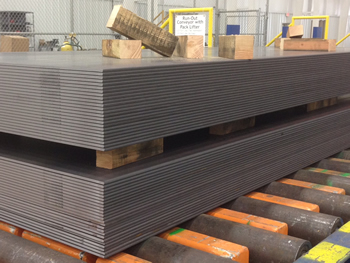### Weight reduction

There’s a rule of thumb for weight reduction where you can make a rough estimate of potential weight reduction by using higher yield steel.

Using the example below, with current thickness being .50” and material 36 KSI min yield. By switching to 100 KSI you could go down to .35”, so 30% less.

Formula:

.50” (current thickness) x √36 KSI (current yield) = .35”
100 KSI (new yield)

Note that this is a rough guideline and all aspects of possible design revision needs to be verified by engineering.

### Cost Analysis

Higher yield material has a higher cost per lb. but since less material is needed it’s still cost efficient. On this example, new material cost is 30% higher and weight reduction is 30%.

• Current product cost: \$ 75/cwt , cost for 1,000 lbs. = \$ 750
• Grade 100 cost ; \$ 95/cwt, cost for 700 lbs. = \$ 665

Payload would increase 300 lbs. for every 1,000 lbs. converted to Grade 100 with lower material cost. There would be also freight savings for incoming freight of steel sheets. On this example freight on every third truckload would be free.